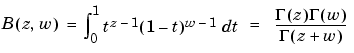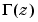MATLAB Function Referencebeta

Beta function

Syntax

• ```B = beta(Z,W)
```

Definition

The beta function is

•whereis the gamma function.

Description

```B = beta(Z,W) ``` computes the beta function for corresponding elements of arrays `Z` and `W`. The arrays must be real and nonnegative. They must be the same size, or either can be scalar.

Examples

In this example, which uses integer arguments,

• ```beta(n`,`3)
= (n-1)!*2!/(n+2)!
= 2/(n*(n+1)*(n+2))
```

is the ratio of fairly small integers, and the rational format is able to recover the exact result.

• ```format rat
beta((0:10)',3)

ans =

1/0
1/3
1/12
1/30
1/60
1/105
1/168
1/252
1/360
1/495
1/660
```

Algorithm

• ``````beta(z,w) = exp(gammaln(z)+gammaln(w)-gammaln(z+w))
``````

See Also

`betainc`, `betaln`, `gammaln`

© 1994-2005 The MathWorks, Inc.